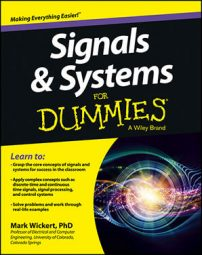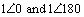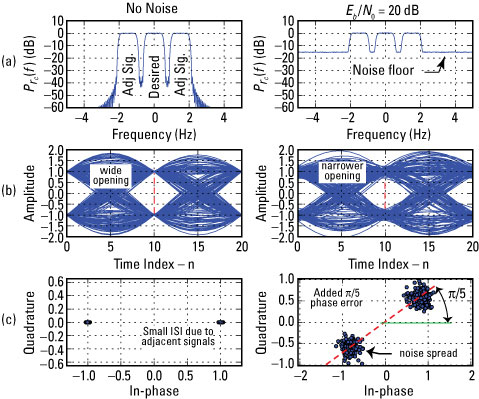##### Signals and Systems For DummiesAs a simple experiment, this case study generates three tightly packed SRC shaped BPSK signals. The signal of interest is centered at f = 0, while the cochannel signals — those that are nearest neighbors — are at offsets of +/– 1.5 Rb Hz. The power level of the adjacent signals is 0 dB relative to the desired signal.

## Check performance with power spectrum, eye, and scatter plots

To characterize the received signal, view the power spectrum at the receiver input, the eye plot of the real part of the matched filter output, and a scatter plot of the full complex envelope at the matched filter output. Keep in mind that the matched filter has impulse response identical to the transmitter pulse shape function.

The eye plot operates at the waveform level, typically observing the output of the matched filter by overlaying integer multiples of the signaling interval (bit period). The period in this simulation is Ns = 10 samples.

For the eye plot, a 20-sample window is used. The signal smoothly transitions between +/– 1 levels according to the transmitted bit pattern. When contiguous waveform slices are overlaid, there’s a point once per symbol where the waveform isn’t transitioning. This is where the symbol decisions are made. Was a +1 or a –1 sent? The region void of transitions appears as an open eye.

The scatter plot collects the complex outputs from the matched filter once per bit (symbol) period. The ideal sample point locations constitute what’s known as the signal constellation. The points are plotted as dots, so you can see the quality of the sample points entering the receiver Symbol Decision block.

For BPSK, you can expect to see two clusters of points: One cluster corresponds to the –1 bit decisions, and the other cluster corresponds to the +1 bit decisions. This is the nature of the BPSK signal constellation: clusters at these degreesIf a phase error goes uncorrected further upstream in the receiver signal processing, the constellation will be rotated relative to the real axis.

Power spectrum, eye, and scatter plots are shown as a collection of six PyLab subplots.Credit: Illustration by Mark Wickert, PhD

Here are the abbreviated IPython commands:

```In [<b>733</b>]: r, b, data0 = ssd.BPSK_tx(100000,10,1.5,0,'src')
# 100000 symbols, Ns=10, Df = 1.5*Rb, 0dB down
In [<b>734</b>]: r = ssd.cpx_AWGN(r,100,10) # EsN0=100dB, Ns=10
In [<b>735</b>]: Pr,f = ssd.psd(r,2**10,Fs=10)
In [<b>737</b>]: plot(f,10*log10(Pr))
In [<b>743</b>]: z = signal.lfilter(b,1,r)# b is the SRC filter
In [<b>748</b>]: ssd.eye_plot(real(z[2000:6000]),20)#20 samp wind
In [<b>750</b>]: ssd.scatter_plot(z[2000:6000],10,0)
In [<b>775</b>]: r, b, data0 = ssd.BPSK_tx(100000,10,1.5,0,'src')
In [<b>776</b>]: r = ssd.cpx_AWGN(r,20,10) # EsN0=20dB, Ns=10
In [<b>777</b>]: Pr,f = ssd.psd(r,2**10,Fs=10)
In [<b>778</b>]: plot(f,10*log10(Pr))
In [<b>784</b>]: z = signal.lfilter(b,1,r)
In [<b>785</b>]: ssd.eye_plot(real(z[2000:6000]),20)#20 samp wind
In [<b>787</b>]: ssd.scatter_plot(z[2000:6000]*exp(1j*pi/5),10,0)```

Even with adjacent signals at +/– 1.5 Rb, there’s little noticeable impact in the eye plot and scatter plot. Note that the bit rate is set to 1.0 for convenience. Yes, the clusters should be pinpoints if the SRC filters are perfect and no ISI occurs. Still, the performance is good for the given spectrum packing.

As the energy per symbol to noise spectral density (Eb / N0) is reduced for 100 dB (essentially no noise) to just 20 dB, the noise is noticeable in the right column of plots. No bit errors are present because the eye plot is still open. The added phase error to the scatter plot still doesn’t alter the decision process.

For BPSK, the decision boundary is the y-axis. The two clusters lie on opposites sides.

## Look for bit errors

You can implement the bit (symbol) decision process at the IPython command line and compare the resulting bits with the transmitted bits returned by ssd.BPSK_tx() to data0. The matched filter output is vector z. To move beyond the pulse shape delay due to the transmitter and receiver processing requires a 2 x 6 = 12 bit delay, or 10 x 12 = 120 samples.

You take samples of the matched filter output with a stride (multiple) of 10 beginning at the maximum eye opening. The stride of 10 is used because this is the number of samples per bit, Ns, used in the simulation. By aligning at the maximum eye opening you are performing manual bit synchronization.

Bit decisions are made by using the real part and then using the sign() function to declare +1 for values greater than zero and –1 for values less than zero. Finally, you shift the hard decision values back to 0/1 values.

```In [<b>850</b>]: data0[0:20]
Out[<b>850</b>]: array([1, 1, 0, 1, 0, 1, 1, 1, 1, 1, 1, 0, 1, 1,
1, 0, 0, 0, 1, 0])
In [<b>851</b>]: int64((sign(real(z[120:320:10]))+1)/2)
Out[<b>851</b>]: array([1, 1, 0, 1, 0, 1, 1, 1, 1, 1, 1, 0, 1, 1,
1, 0, 0, 0, 1, 0])```

For the 20 bits compared, all is well! By XORing (using the Python operator ^) trimmed versions of the two vectors, you can count bit errors and estimate the probability of bit error, Pe, by dividing by the total number of bits in the comparison. Checking the first 10,000 bits yields the following code:

```In [<b>862</b>]: sum(data0[0:10000]^int64((sign(real(z[120:(120+10000*10):10]))+1)/2))/float(len(data0[0:10000]))
Out[<b>862</b>]: 0.0 # Pe_est```

No errors counted, so the estimated bit error probability is zero. Are you surprised? For further study, decrease (Eb / N0)dB until bit errors start to appear.